wwwtyro.net Go back Open original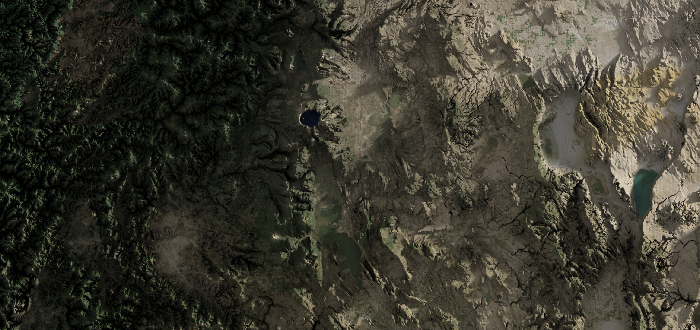There’s only one common means of adding shading to maps today - hillshading. It’s a simple and effective way to add a sense of relief to maps, but we can do better. This post will cover the basics of hillshading, then move to the more advanced topics of soft shadows and ambient lighting. To lend speed, we’ll perform these calculations on the GPU in WebGL with the incomprehensibly good regl library.

## Prerequisite: Getting Tiles From A Tile Server

Source

For this post, I’ll be using the mapbox service to load tiles. Another good option is maptiler. The interface for these services are all quite similar, so you can probably use nearly-identical code for the service of your choice. Here’s what it looks like at a high level.

First we’ll require some utility functions (more on those in a moment):

``````const demo = require("./demo");
``````

Then we’ll load up our mapbox key (I’m using Browserify and brfs to make the following work at build time):

``````const fs = require("fs");
``````

Next we’ll define a zoom and a latitude/longitude location. I’ve chosen a point along the Colorado River running through the Grand Canyon:

``````async function main() { const zoom = 10; const [lat, long] = [36.133487, -112.239884];
``````

Then we’ll convert those into tile coordinates. I’ll cover the `lat2tile` and `long2tile` functions in a moment, but understand that those functions return `x` & `y` coordinates that are floats, not integers. We’ll need integers to interact with the tile server, and we’ll want to round down to get the tile the floating point coordinates are in.

`````` const tLat = Math.floor(demo.lat2tile(lat, zoom)); const tLong = Math.floor(demo.long2tile(long, zoom));
``````

`````` const image = await demo.loadImage( `https://api.mapbox.com/v4/mapbox.satellite/\${zoom}/\${tLong}/\${tLat}.pngraw?access_token=\${MAPBOX_KEY}` );
``````

And finally display it on the page:

`````` document.body.appendChild(image);
} main();
``````

Now let’s look at those utility functions in the `demo` module. First, the functions that turn latitude and longitude into tile coordinates. We won’t go into detail here, but you can get more information about them at this OpenStreetMap wiki page.

``````function long2tile(l, zoom) { return ((l + 180) / 360) * Math.pow(2, zoom);
} function lat2tile(l, zoom) { return ( ((1 - Math.log( Math.tan((l * Math.PI) / 180) + 1 / Math.cos((l * Math.PI) / 180) ) / Math.PI) / 2) * Math.pow(2, zoom) );
}
``````

Finally, the `loadImage` function is just a wrapper around the `Image` object that returns a promise that we can `await`:

``````function loadImage(url) { console.log(`Downloading \${url}...`); return new Promise((accept, error) => { const img = new Image(); img.onload = () => { accept(img); }; img.onerror = error; img.crossOrigin = "anonymous"; img.src = url; });
}
``````

The result of all that looks like this: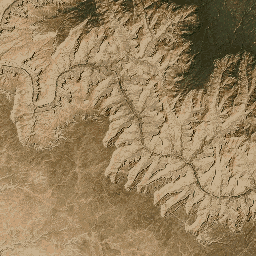A satellite imagery tile loaded from the mapbox service.

Hillshading is simply direct lighting with no shadows. We only need two pieces of information for direct lighting: the normal at each pixel and the direction of the light source. Some tile services provide surface normal tiles, but some don’t, so we’ll proceed assuming we only have elevation information. We’ll need elevation for our advanced shading anyway, so win-win!

### Elevation

Source

We’ll gather our elevation data from mapbox terrain-rgb tiles. These are just PNGs that encode the elevation in the red, green, and blue channels. We’ll decode the elevation and scale it by whatever factor we choose.

First we’ll `require` the regl library, which we’ll be using to interact with WebGL.

``````const REGL = require("regl");
``````

As before, we’ll grab the tile from mapbox, but this time we’ll use the `mapbox.terrain-rgb` endpoint. Note: starting here I’ll be excluding repetitive portions of the code. See the source to recover the full picture.

`````` const image = await demo.loadImage( `https://api.mapbox.com/v4/mapbox.terrain-rgb/\${zoom}/\${tLong}/\${tLat}.pngraw?access_token=\${MAPBOX_KEY}` );
``````

Unprocessed, that image looks like this: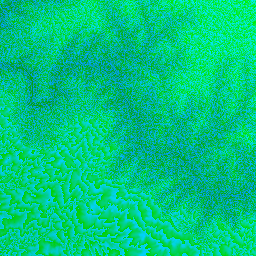A terrain-rgb tile loaded from the mapbox service.

Let’s create a canvas, size it according to the dimensions of the tile, and append it to the page:

`````` const canvas = document.createElement("canvas"); canvas.width = image.width; canvas.height = image.height; document.body.appendChild(canvas);
``````

Next we’ll create the regl context:

`````` const regl = REGL({ canvas: canvas });
``````

Then we’ll create a regl texture object using the terrain-rgb tile image we just downloaded. We’ll also flip it vertically so that we match WebGL conventions:

`````` const tElevation = regl.texture({ data: image, flipY: true });
``````

Next we’ll create the regl command that we’ll use to process the elevation. There’s a couple things to note here. First, we’re rendering to a quad that completely fills the viewport (see the `attributes.position` section). Second, we’re adding a uniform that will allow us to scale the elevation. We’ll use different values later, but for now we’ll find a value (`0.0005`) that shows reasonable contrast when displayed, instead of washing everything out in white.

`````` const cmdProcessElevation = regl({ vert: ` precision highp float; attribute vec2 position; void main() { gl_Position = vec4(position, 0, 1); } `, frag: ` precision highp float; uniform sampler2D tElevation; uniform vec2 resolution; uniform float elevationScale; void main() { // Sample the terrain-rgb tile at the current fragment location. vec3 rgb = texture2D(tElevation, gl_FragCoord.xy/resolution).rgb; // Convert the red, green, and blue channels into an elevation. float e = -10000.0 + ((rgb.r * 255.0 * 256.0 * 256.0 + rgb.g * 255.0 * 256.0 + rgb.b * 255.0) * 0.1); // Scale the elevation and write it out. gl_FragColor = vec4(vec3(e * elevationScale), 1.0); } `, attributes: { position: [-1, -1, 1, -1, 1, 1, -1, -1, 1, 1, -1, 1] }, uniforms: { tElevation: tElevation, elevationScale: 0.0005, resolution: [image.width, image.height] }, viewport: { x: 0, y: 0, width: image.width, height: image.height }, count: 6 });
``````

Finally, we’ll invoke the regl command we just wrote:

Which results in the following: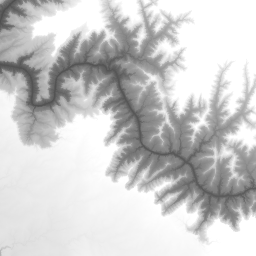The terrain-rgb tile decoded into elevation. Darker pixels represent a lower elevation.

### Normals

Source

Now that we have our elevation data, we’re ready to calculate a normal map. First though, we’ll need to make a couple minor alterations to how we store the elevation data. We’ll want to store the elevation in a framebuffer, and we’ll want that framebuffer to be backed by a floating point texture. In order to use floating point textures in WebGL 1.0, we need to use the `OES_texture_float` extension. Here’s how that’s done in regl:

`````` // Create the regl context. const regl = REGL({ canvas: canvas, extensions: ["OES_texture_float"] });
``````

Once we have the extension, we can create a floating point regl framebuffer to store the elevation data:

`````` const fboElevation = regl.framebuffer({ width: image.width, height: image.height, colorType: "float" });
``````

Then in the `cmdProcessElevation` regl command, we’ll set the elevation scale to `1.0`:

And set the target framebuffer to the one we just made:

`````` framebuffer: fboElevation,
``````

Our current units are a bit mixed up. The `x-` and `y-` coordinates still represent pixels, but the elevation is stored in meters. We need some conversion that can take us from pixels to meters. This isn’t something we can solve for the entire tile - that scale would vary from pixel to pixel because of the Mercator projection that is flattening the map. Here, we’ll approximate it for all pixels in the tile by figuring out the longitudinal width of the tile, converting that to an arc length, and then dividing that arc length by the number of the pixels in the tile:

`````` long0 = demo.tile2long(tLong, zoom); long1 = demo.tile2long(tLong + 1, zoom); const pixelScale = (6371000 * (long1 - long0) * 2 * Math.PI) / 360 / image.width;
``````

Next we’ll create a regl command that will calculate a normal for each pixel. There’s a lot of ways to do this, but here’s what we’ll do: we’ll grab the elevation of the current pixel, the elevation of one pixel north, and the elevation of one pixel east. We’ll then construct two vectors - one that points from the current pixel to the one north, and one that points from our current pixel to the one east. These are 3D vectors that incorporate the `pixelScale` we just calculated and the elevation. Once we have those two vectors, we can take their cross product and normalize it to create a normal vector for our current pixel.

The components of this normal vector exist on the range . To display them, we’ll convert them to the range .

Note that the normals at the positive `x-` and `y-` edges of the map are ill defined - there isn’t any data there about the pixels to the north and east! I’ll address that later, but for now know that if you tried to render a tiled map like this, you’d likely see artifacts at the edges of the tiles.

`````` const cmdNormal = regl({ vert: ` precision highp float; attribute vec2 position; void main() { gl_Position = vec4(position, 0, 1); } `, frag: ` precision highp float; uniform sampler2D tElevation; uniform vec2 resolution; uniform float pixelScale; void main() { vec2 dr = 1.0/resolution; float p0 = texture2D(tElevation, dr * (gl_FragCoord.xy + vec2(0.0, 0.0))).r; float px = texture2D(tElevation, dr * (gl_FragCoord.xy + vec2(1.0, 0.0))).r; float py = texture2D(tElevation, dr * (gl_FragCoord.xy + vec2(0.0, 1.0))).r; vec3 dx = vec3(pixelScale, 0.0, px - p0); vec3 dy = vec3(0.0, pixelScale, py - p0); vec3 n = normalize(cross(dx, dy)); gl_FragColor = vec4(0.5 * n + 0.5, 1.0); } `, attributes: { position: [-1, -1, 1, -1, 1, 1, -1, -1, 1, 1, -1, 1] }, uniforms: { tElevation: fboElevation, pixelScale: pixelScale, resolution: [image.width, image.height] }, viewport: { x: 0, y: 0, width: image.width, height: image.height }, count: 6 });
``````

Finally, we’ll invoke our new regl command:

Which will result in a normal map: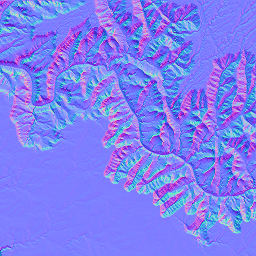The normal map constructed from the elevation data.

Lastly, here’s the `tile2long` function we invoked earlier:

``````function tile2long(x, z) { return (x / Math.pow(2, z)) * 360 - 180;
}
``````

### Direct Lighting

Source

Now that we have a normal map for our terrain, we can pretty easily calculate direct lighting without shadows, or hillshading. First, a few minor details.

We’ll want to define a direction for the sun later, and we’ll want that direction to be normalized. We’ll `require` the `vec3` module of the `gl-matrix` library to make that normalization convenient.

``````const { vec3 } = require("gl-matrix");
``````

As before, we’ll want to store our normals in a floating point framebuffer. Let’s make that framebuffer:

`````` const fboNormal = regl.framebuffer({ width: image.width, height: image.height, colorType: "float" });
``````

When we displayed the normals before, we transformed them to be on the range . We’ll just write out the untransformed normal in the `cmdNormals` regl command now:

`````` gl_FragColor = vec4(n, 1.0);
``````

And we’ll write them to the framebuffer we just created:

We’ll define the sun direction to be the normalized vector. This will place it equally north, east, and above our terrain. We’ll grab the normal for each pixel and take the dot product of that with the normalized sun direction. The result will be a light intensity that varies on the range .

Values less than zero are simply black, so we’re throwing away half of the information about our surface. Instead, we’ll transform the light intensity to the range to achieve a nice shading effect. This is an artistic choice - feel free to play with this transform to reach whatever lighting you’d like.

Here’s all that bottled up into our new regl command:

`````` const cmdDirect = regl({ vert: ` precision highp float; attribute vec2 position; void main() { gl_Position = vec4(position, 0, 1); } `, frag: ` precision highp float; uniform sampler2D tNormal; uniform vec2 resolution; uniform vec3 sunDirection; void main() { vec2 dr = 1.0/resolution; vec3 n = texture2D(tNormal, gl_FragCoord.xy/resolution).rgb; float l = dot(n, sunDirection); l = 0.5 * l + 0.5; gl_FragColor = vec4(l, l, l, 1.0); } `, attributes: { position: [-1, -1, 1, -1, 1, 1, -1, -1, 1, 1, -1, 1] }, uniforms: { tNormal: fboNormal, resolution: [image.width, image.height], sunDirection: vec3.normalize([], [1, 1, 1]) }, viewport: { x: 0, y: 0, width: image.width, height: image.height }, count: 6 });
``````

Finally, we’ll invoke our new command:

Which results in a nice hillshaded terrain: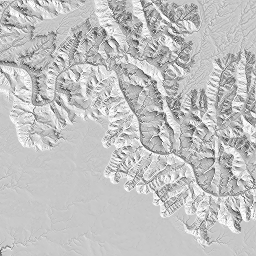Source

Note: For this and following sections, I scale the terrain by a factor of four to increase the contrast of the shading effects. This is an artistic choice - you should play with this value to find an appearance appealing to you.

Real lights, like the sun, have some size, they aren’t infinitesimally small. This is why real lights create soft shadows. Consider the following figure:Soft shadows manifest because real lights have a size.

From the perspective of point A, the entire light can be seen. From B, only a portion of the light can be seen, and C can see none of the light because it is completely blocked by an occluder. Imagine moving from point A to point C. Initially, you see the entire light, then the occluder blocks a tiny portion of the light, then more and more of the light is blocked until you can see none of the light and are completely in shadow. It is this transition that produces soft shadows.

Additionally, the size of the light impacts the magnitude of the soft shadowing. The larger the light, the softer the shadows. If you think about it in terms of the transition from A to C we just described, it makes sense - it takes longer to transition from a fully visible light to a fully occluded light.

To calculate our soft shadows, we’re going to cast a ray from each pixel into some random point on the sun, multiple times, and average over the illumination for each ray. When we’re situated at point A, all of our rays will strike the sun. At B, some will hit and some will hit the occluder. At C, all will hit the occluder, and none will hit the sun. The average over all these hits and misses yields the value for our soft shadows.

Let’s talk about how to cast rays.

### Fast Pixel Traversal

John Amanatides and Andrew Woo published “A Fast Voxel Traversal Algorithm for Ray Tracing” in 1987. It’s one of my favorite papers because of its accessibility and relevance to my interests. I’ll summarize it here in the context of our 2D application.

This algorithm is composed of two phases: initialization and traversal. Let’s start with initialization.

First, we find the pixel we’re starting in (`p` below). Then we find the sign of our ray direction along each axis (`stp`). Next we find how far we need to travel in our ray direction to intersect the next pixel in the `x-` and `y-` directions (`tMax`). Finally, we determine how far we must travel along our ray to cover the width and height of a pixel (`tDelta`).

At this point we can begin the traversal phase of the algorithm. Here’s what it looks like in pseudocode:

``````while (true) { if (tMax.x < tMax.y) { tMax.x += tDelta.x p.x += stp.x } else { tMax.y += tDelta.y p.y += stp.y } if (exitedTile() or hitTerrain()) { break }
}
``````

Isn’t that wonderfully simple? This allows us to traverse our texture and not skip over or miss any pixels.

### Ping-pong Technique

The way we’ll average all those shadow rays is by using the ping-pong technique. We’ll render to a destination framebuffer, then use it as a source texture in our next iteration. Then we’ll swap them again and continue. We’ll be rendering a fixed number of times (let’s call it ), so each iteration we’ll add to the illumination we accumulate. When we’re done, the last framebuffer we rendered to contains our final averaged result. The `demo` module has a convenience function to wrap all this up for us:

``````function PingPong(regl, opts) { const fbos = [regl.framebuffer(opts), regl.framebuffer(opts)]; let index = 0; function ping() { return fbos[index]; } function pong() { return fbos[1 - index]; } function swap() { index = 1 - index; } return { ping, pong, swap };
}
``````

### Implementation

Now we’re ready to begin. First we’ll create our ping-pong framebuffers:

``````const shadowPP = demo.PingPong(regl, { width: image.width, height: image.height, colorType: "float"
});
``````

Next we’ll write a regl command that will calculate the illumination of a single ray and add it to the result of the last iteration. The vertex shader remains the same:

``````const cmdSoftShadows = regl({ vert: ` precision highp float; attribute vec2 position; void main() { gl_Position = vec4(position, 0, 1); } `,
``````

Now we can start our fragment shader:

`````` frag: ` precision highp float; uniform sampler2D tElevation; uniform sampler2D tNormal; uniform sampler2D tSrc; uniform vec3 sunDirection; uniform vec2 resolution; uniform float pixelScale; void main() { vec2 ires = 1.0 / resolution;
``````

We’ll grab the normal and elevation from their respective textures, and the illumination from the previous iteration (`src`).

`````` vec3 src = texture2D(tSrc, gl_FragCoord.xy * ires).rgb; vec4 e0 = texture2D(tElevation, gl_FragCoord.xy * ires); vec3 n0 = texture2D(tNormal, gl_FragCoord.xy * ires).rgb;
``````

Then we’ll normalize the two dimensional component of the direction to the sun to get our 2D ray direction `sr`.

`````` vec2 sr = normalize(sunDirection.xy);
``````

Then we’ll initialize the pixel traversal algorithm as described above:

`````` vec2 p0 = gl_FragCoord.xy; vec2 p = floor(p0); vec2 stp = sign(sr); vec2 tMax = step(0.0, sr) * (1.0 - fract(p0)) + (1.0 - step(0.0, sr)) * fract(p0); tMax /= abs(sr); vec2 tDelta = 1.0 / abs(sr);
``````

And begin stepping:

`````` for (int i = 0; i < 65536; i++) { if (tMax.x < tMax.y) { tMax.x += tDelta.x; p.x += stp.x; } else { tMax.y += tDelta.y; p.y += stp.y; }
``````

At each step we’ll grab the normalized texture coordinate for the center of the current pixel and check to see if we’ve left the bounds of the tile. If we have, we’ll add some illumination to the pixel for this iteration and stop iterating. I’m performing 128 iterations, which is where the comes from. The dot product takes into account the angle of illumination. Previously we transformed this result to the range , but here we’ll clamp it to that range.

`````` vec2 ptex = ires * (p + 0.5); if (ptex.x < 0.0 || ptex.x > 1.0 || ptex.y < 0.0 || ptex.y > 1.0) { gl_FragColor = vec4(src + vec3(1.0/128.0) * clamp(dot(n0, sunDirection), 0.0, 1.0), 1.0); return; }
``````

If we didn’t exit the tile, we’ll need to see if we hit any terrain. Let’s get the elevation at the current pixel:

`````` vec4 e = texture2D(tElevation, ptex);
``````

We’ll calculate the time (`t`) we’ve traveled along the 2D ray and use that to determine how far up we’ve moved from our starting point to recover an elevation at the current point along our original 3D ray:

`````` float t = distance(p + 0.5, p0); float z = e0.r + t * pixelScale * sunDirection.z;
``````

If the elevation of the pixel we’re on is greater than the elevation we’re at along our 3D ray, we’ve hit terrain. We’ll store the illumination from the previous iteration, effectively adding a zero for this iteration:

`````` if (e.r > z) { gl_FragColor = vec4(src, 1.0); return; }
``````

We set up our loop to iterate many more times than we needed to traverse the tile, so we should never hit this case, but if we finish the loop, let’s pretend it’s been illuminated. You could also render a unique color here to try to debug this case:

`````` } gl_FragColor = vec4(src + vec3(1.0/128.0) * clamp(dot(n0, sunDirection), 0.0, 1.0), 1.0); } `,
``````

There’s a couple more things of note in this regl command. We disable the depth buffer to make sure we can write each time, and we add regl props (parameters, essentially) for the `sunDirection` and source and destination framebuffers (`src` and `dest`, respectively).

`````` depth: { enable: false }, attributes: { position: [-1, -1, 1, -1, 1, 1, -1, -1, 1, 1, -1, 1] }, uniforms: { tElevation: fboElevation, tNormal: fboNormal, tSrc: regl.prop("src"), sunDirection: regl.prop("sunDirection"), pixelScale: pixelScale, resolution: [image.width, image.height] }, viewport: { x: 0, y: 0, width: image.width, height: image.height }, framebuffer: regl.prop("dest"), count: 6
});
``````

Now that our command is complete, we’ll invoke it 128 times (recall the normalization constant earlier). Each time we invoke it, we’ll swap the ping-pong framebuffers and calculate a new sun direction. The `100` below indicates a multiple of sun radii, so we’re using a fake sun that is 100 times larger (in terms of its radius) than the real sun.

On the last frame (`i === 127`), we’ll render to the screen instead of the framebuffer.

``````for (let i = 0; i < 128; i++) { cmdSoftShadows({ sunDirection: vec3.normalize( [], vec3.add( [], vec3.scale([], vec3.normalize([], [1, 1, 1]), 149600000000), vec3.random([], 695508000 * 100) ) ), src: shadowPP.ping(), dest: i === 127 ? undefined : shadowPP.pong() }); shadowPP.swap();
}
``````

The result is the following: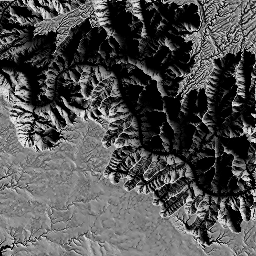Here’s a comparison to hard shadows (rendered by changing the `100` mentioned above to `0`):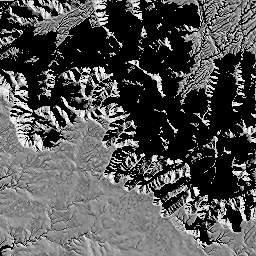## Ambient Lighting

Source

Ambient light is the sum of all light reaching a surface, minus direct light. In the context of our map application, you’d consider this the light hitting the map from the sky, but not the sun. Importantly, this light is occluded by nearby terrain, so you’d expect the amount of ambient light hitting a plateau to be rather high, while the amount of ambient light hitting a deep crevice should be low.

Calculating the amount of ambient light is very nearly identical to the lighting calculation performed for soft shadows. The primary exception is that instead of shooting rays towards the sun, we simply shoot them randomly off the surface and see if they hit the terrain. If they don’t, we add to the illumination, if they do, we don’t. Let’s take a look.

Since we’re taking an average again, we’ll also create ping-pong framebuffers here:

``````const ambientPP = demo.PingPong(regl, { width: image.width, height: image.height, colorType: "float"
});
``````

The regl command is nearly identical, with the exceptions highlighted below.

``````const cmdAmbient = regl({ vert: ` precision highp float; attribute vec2 position; void main() { gl_Position = vec4(position, 0, 1); } `, frag: ` precision highp float; uniform sampler2D tElevation; uniform sampler2D tNormal; uniform sampler2D tSrc; uniform vec3 direction; uniform vec2 resolution; uniform float pixelScale; void main() { vec2 ires = 1.0 / resolution; vec3 src = texture2D(tSrc, gl_FragCoord.xy * ires).rgb; vec4 e0 = texture2D(tElevation, gl_FragCoord.xy * ires); vec3 n0 = texture2D(tNormal, gl_FragCoord.xy * ires).rgb;
``````

Here’s how we’ll calculate our ray direction: imagine a sphere of radius one meter sitting on the surface at the current pixel. Select a point at random from the volume of the sphere. Create a vector from the surface point to the random point and normalize it. That’s the ray direction. The reason we’re doing it this way is to generate a distribution of rays that closely matches how rays bounce off of a completely diffuse surface.

Turns out this is super simple in code:

`````` vec3 sr3d = normalize(n0 + direction);
``````

The rest of the regl command is pretty much identical, except that we don’t scale the illumination by the dot product of the surface normal with the ray direction - that’s already taken care of by the distribution of rays we’re generating.

`````` vec2 sr = normalize(sr3d.xy); vec2 p0 = gl_FragCoord.xy; vec2 p = floor(p0); vec2 stp = sign(sr); vec2 tMax = step(0.0, sr) * (1.0 - fract(p0)) + (1.0 - step(0.0, sr)) * fract(p0); tMax /= abs(sr); vec2 tDelta = 1.0 / abs(sr); for (int i = 0; i < 65536; i++) { if (tMax.x < tMax.y) { tMax.x += tDelta.x; p.x += stp.x; } else { tMax.y += tDelta.y; p.y += stp.y; } vec2 ptex = ires * (p + 0.5); if (ptex.x < 0.0 || ptex.x > 1.0 || ptex.y < 0.0 || ptex.y > 1.0) { gl_FragColor = vec4(src + vec3(1.0/128.0), 1.0); return; } vec4 e = texture2D(tElevation, ptex); float t = distance(p + 0.5, p0); float z = e0.r + t * pixelScale * sr3d.z; if (e.r > z) { gl_FragColor = vec4(src, 1.0); return; } } gl_FragColor = vec4(src + vec3(1.0/128.0), 1.0); } `, depth: { enable: false }, attributes: { position: [-1, -1, 1, -1, 1, 1, -1, -1, 1, 1, -1, 1] }, uniforms: { tElevation: fboElevation, tNormal: fboNormal, tSrc: regl.prop("src"), direction: regl.prop("direction"), pixelScale: pixelScale, resolution: [image.width, image.height] }, viewport: { x: 0, y: 0, width: image.width, height: image.height }, framebuffer: regl.prop("dest"), count: 6
});
``````

Finally, the random vectors we provide are of a random length within the unit sphere:

``````for (let i = 0; i < 128; i++) { cmdAmbient({ direction: vec3.random([], Math.random()), src: ambientPP.ping(), dest: i === 127 ? undefined : ambientPP.pong() }); ambientPP.swap();
}
``````

Here’s the result: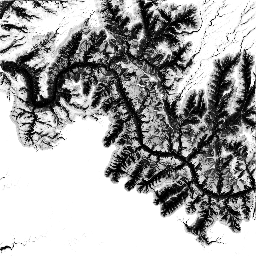Ambient lighting.

## Combined Lighting

Source

Now that we have both of the components of lighting, we can simply add them together to create the final light map.

Before we do that, let’s change the following lines in `cmdSoftShadow` and `cmdAmbient`:

`````` dest: i === 127 ? undefined : shadowPP.pong()
``````
`````` dest: i === 127 ? undefined : ambientPP.pong()
``````

to the following:

This will allow us to store the component of each within the `ping()` value of the ping-pong framebuffers. Note that while the last destination is `pong()`, there’s a final swap that occurs at the end of the loop.

Adding up the lighting is unsurprisingly simple. The only twist is that we multiply each component by some factor, in this case `1.0` for the soft shadow lighting and `0.25` for the ambient light. This is again an artistic choice - tweak this to your heart’s desire.

``````const cmdFinal = regl({ vert: ` precision highp float; attribute vec2 position; void main() { gl_Position = vec4(position, 0, 1); } `, frag: ` precision highp float; uniform sampler2D tSoftShadow; uniform sampler2D tAmbient; uniform vec2 resolution; void main() { vec2 ires = 1.0 / resolution; float softShadow = texture2D(tSoftShadow, ires * gl_FragCoord.xy).r; float ambient = texture2D(tAmbient, ires * gl_FragCoord.xy).r; float l = 1.0 * softShadow + 0.25 * ambient; gl_FragColor = vec4(l,l,l, 1.0); } `, depth: { enable: false }, attributes: { position: [-1, -1, 1, -1, 1, 1, -1, -1, 1, 1, -1, 1] }, uniforms: { tSoftShadow: shadowPP.ping(), tAmbient: ambientPP.ping(), resolution: [image.width, image.height] }, viewport: { x: 0, y: 0, width: image.width, height: image.height }, count: 6
}); cmdFinal();
``````

Here’s the result: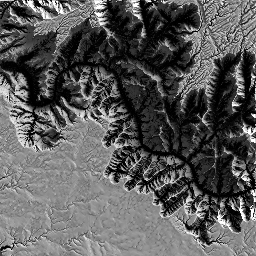Soft shadows and ambient lighting combined.

For the curious, here’s the same combination, but this time with a sun radius multiplier of one: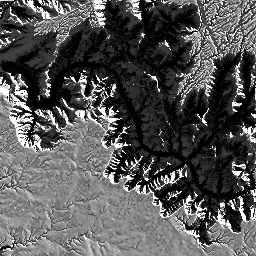Source

We can add arbitrary base colors to apply our lighting to. Let’s try adding satellite imagery.

Note: Satellite imagery comes with baked-in lighting already - from the sun! If you zoom in close enough, you can usually see shadows. To my knowledge, there’s not yet a great way to address this straightforwardly (though there are some satellite shadow-removal papers floating around). The easiest options appear to be zooming out or using a different imagery set.

Let’s download a satellite tile from mapbox and create a texture with it:

``````const satelliteImage = await demo.loadImage( `https://api.mapbox.com/v4/mapbox.satellite/\${zoom}/\${tLong}/\${tLat}.pngraw?access_token=\${MAPBOX_KEY}`
); const tSatellite = regl.texture({ data: satelliteImage, flipY: true
});
``````

In our fragment shader, we’ll pull in the satellite texture:

`````` uniform sampler2D tSatellite;
``````

We’ll grab the texture color:

`````` vec3 satellite = texture2D(tSatellite, ires * gl_FragCoord.xy).rgb;
``````

Tweak our lighting a bit:

`````` float l = 4.0 * softShadow + 0.25 * ambient;
``````

Deepen the satellite texture’s color a bit by applying a curve, and multiply it by the light:

`````` vec3 color = l * pow(satellite, vec3(2.0));
``````

And finally apply gamma correction and display the result:

`````` color = pow(color, vec3(1.0/2.2)); gl_FragColor = vec4(color, 1.0);
``````

Here’s what it looks like with a sun radius multiplier of 100: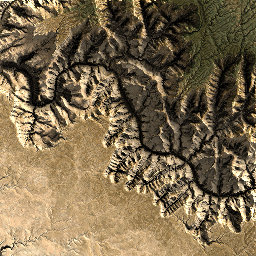Soft shadows and ambient lighting combined with satellite imagery, sun radius multiplier of 100.

And with a sun radius multiplier of one: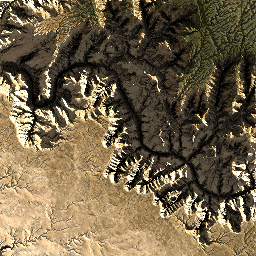Soft shadows and ambient lighting combined with satellite imagery, sun radius multiplier of one.

Of course, you can use whatever map style you have at your disposal: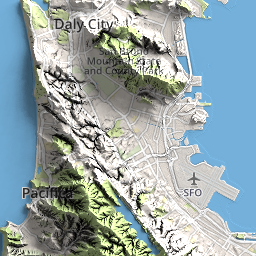Soft shadows and ambient lighting combined with mapbox street tiles, sun radius multiplier of 100.

## Tiling

Source

These are two neighboring tiles: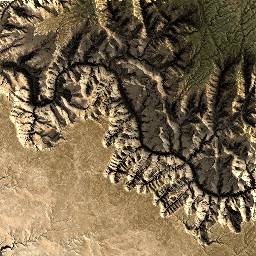Two independently-rendered tiles.

Individually, they look fine. If you display two of these tiles side-by-side, however, some artifacts start appearing: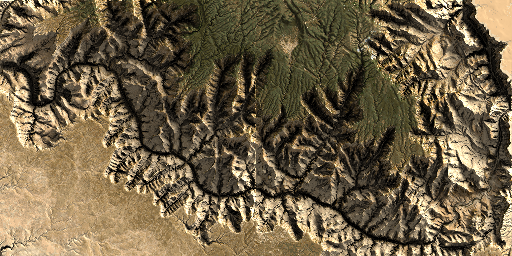The same two tiles, but side-by-side, highlighting the seam at the interface between them.

Ouch.

There’s a couple of problems here. First, as mentioned before, the normals are ill-defined at the positive edges of each tile. Second, the lighting for soft shadows and ambient lighting suffer the same issue, but it’s worse because they need a lot more information than just an additional pixel at the positive edges of the tile - they need to be able to see anything that may occlude them from the sun or sky!

The simple, brute force way of attacking this issue is to simply render your target tile and the eight tiles around it, then extract the target tile. Let’s look at how that’s done, then we’ll discuss some gotchas and optimizations.

First, let’s write a function that returns a `Canvas` object that contains our target tile in the center and the eight surrounding tiles:

``````async function getRegion(tLat, tLong, zoom, api) { const canvas = document.createElement("canvas"); canvas.width = 3 * 256; canvas.height = 3 * 256; const ctx = canvas.getContext("2d"); for (let x = 0; x < 3; x++) { const _tLong = tLong + (x - 1); for (let y = 0; y < 3; y++) { const _tLat = tLat + (y - 1); const url = api .replace("zoom", zoom) .replace("tLat", _tLat) .replace("tLong", _tLong); const img = await loadImage(url); ctx.drawImage(img, x * 256, y * 256); } } return canvas;
}
``````

Now let’s invoke it to grab the elevation:

``````const image = await demo.getRegion( tLat, tLong, zoom, `https://api.mapbox.com/v4/mapbox.terrain-rgb/zoom/tLong/tLat.pngraw?access_token=\${MAPBOX_KEY}`
);
``````

Which looks like this: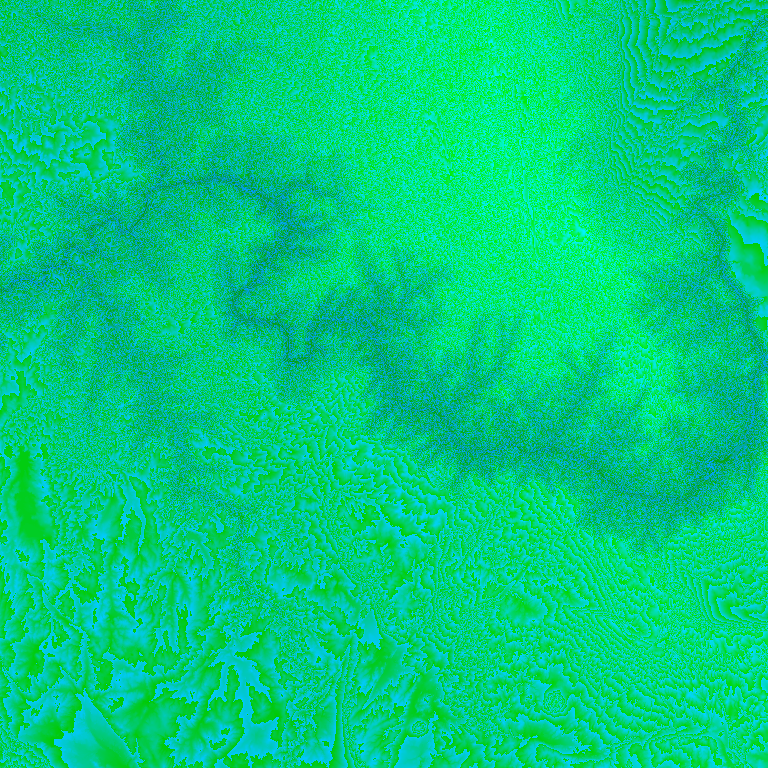Target terrain-rgb tile with its eight neighbors in a single image.

Next, we need to change the way we’re calculating `pixelScale` so that it takes into account the new longitude bounds:

``````long0 = demo.tile2long(tLong - 1, zoom);
long1 = demo.tile2long(tLong + 2, zoom);
const pixelScale = (6371000 * (long1 - long0) * 2 * Math.PI) / 360 / image.width;
``````

And of course we need the large satellite image as well:

``````const satelliteImage = await demo.getRegion( tLat, tLong, zoom, `https://api.mapbox.com/v4/mapbox.satellite/zoom/tLong/tLat.pngraw?access_token=\${MAPBOX_KEY}`
);
``````

Now we render the same two target tiles as before, but with their neighboring tiles, then extract the center from each. First the left: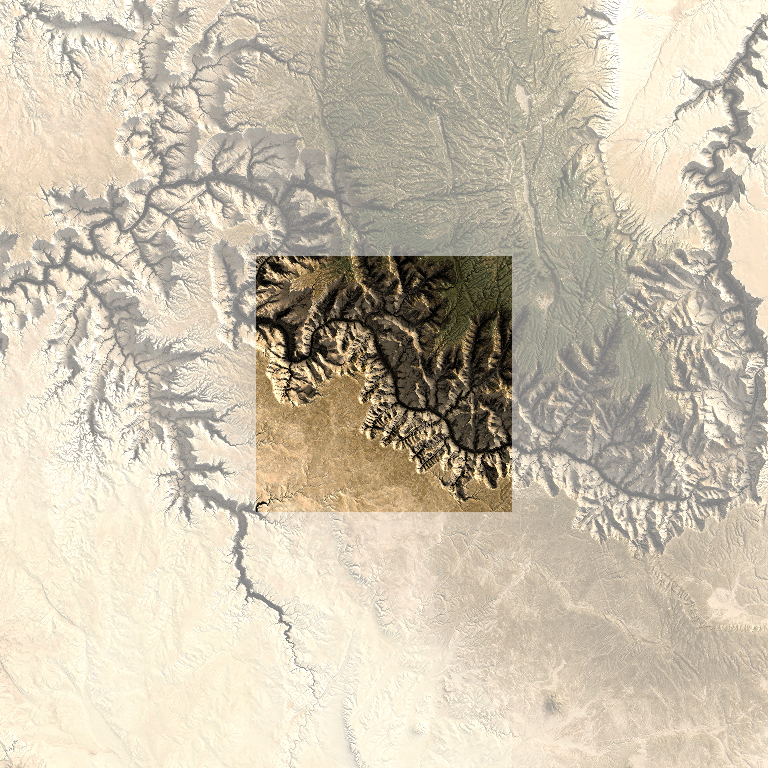Extracting the left tile.

Then the right: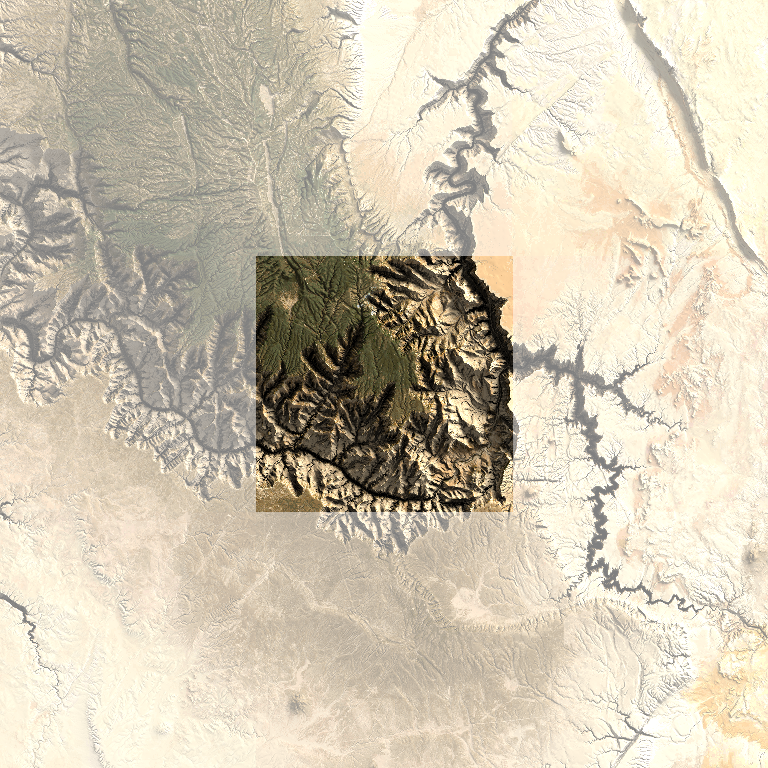Extracting the right tile.

Now we can display the two rendered tiles side-by-side without significant artifacts: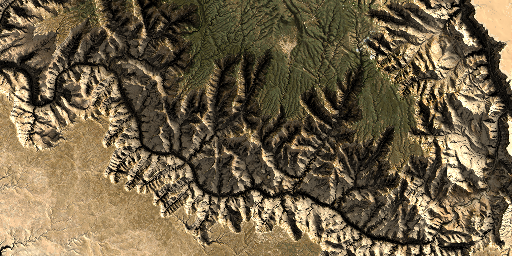Displaying the extracted tiles side-by-side.

### Tiling Gotchas

If your shadows or ambient lighting effects stretch more than a tile away, they could create artifacts. I can imagine a few of ways you might address this:

• For shadows exhibiting this issue, you might raise the light source so that they don’t stretch as far.
• You can increase the radius of the tiles you render until they encompass the region impacted by shadows and ambient lighting.
• You can fade the intensity of shadows and occlusion over the radius of tiles you’re rendering, forcing them to zero before the tile edge. This may result in “square” shadows and occlusion, but probably beats sudden dropoffs in intensity.

If you’re using a low number of samples when averaging over the soft shadows and ambient lighting, you may see artifacts arise from the different distribution of samples per tile. This is easily addressed: create a list of random vectors, then reuse them for every tile, instead of generating a new set for each tile.

I haven’t tried it out yet, but dealing with zoom functionality could be tricky. We won’t be able to calculate the lighting at a high zoom and then construct the images for lower zoom levels by merging the images. After a couple of zoom levels, the effects of shadows and ambient lighting will dissipate because they will be small relative to the map size. We might try to recalculate the lighting at each zoom level, increasing or decreasing the elevation scale as appropriate to make the shading effects scale.

### Tiling Optimizations

• We’re calculating the lighting for all the pixels in all the tiles. There’s no need for that. Just calculate it for the pixels in the center tile.
• Cache all tiles that make sense to cache. There’s no need to recalculate elevation multiple times for a tile, and you certainly shouldn’t be downloading it multiple times.

## Final Notes

To run the demos, you’ll need to provide your own mapbox key. Once you’ve created an account and obtained a key, drop it into a file named `mapbox.key` in the root of the demo repo.

You can of course use this technique on 3D meshes as well. You just need to generate a mesh with proper UV coordinates and apply the texture you generated. I’ve included some sample images at the end of this post demonstrating it.

I’ve generated maps for all 50 states and DC. They are available here. Enjoy.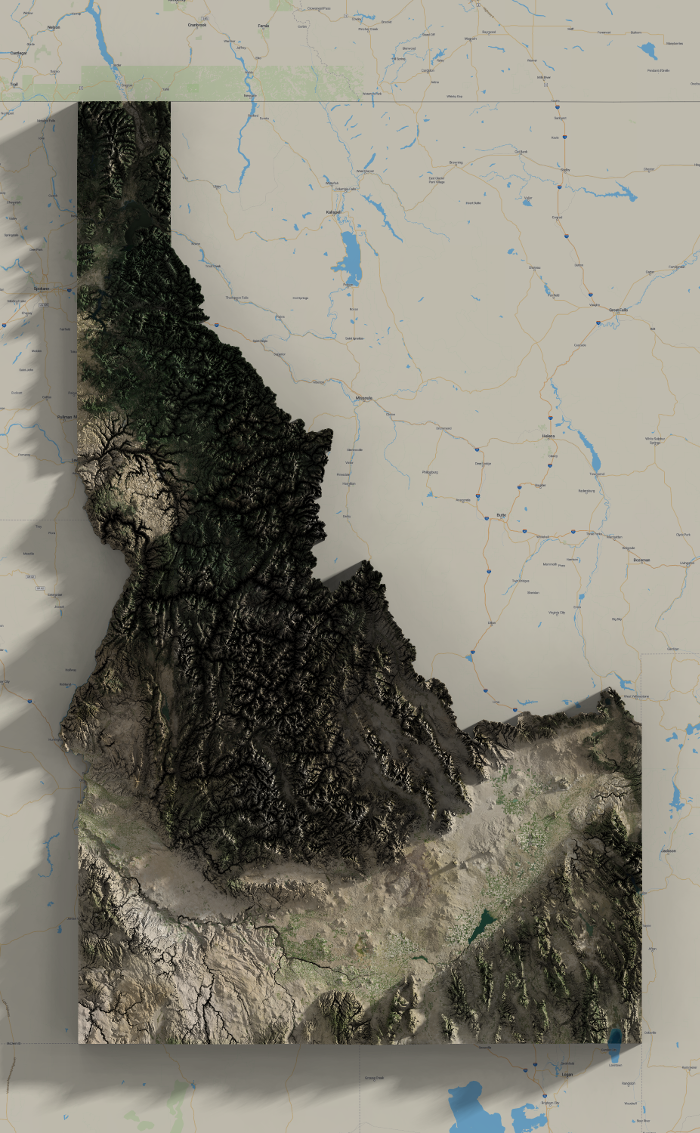Idaho has some wonderful contrast.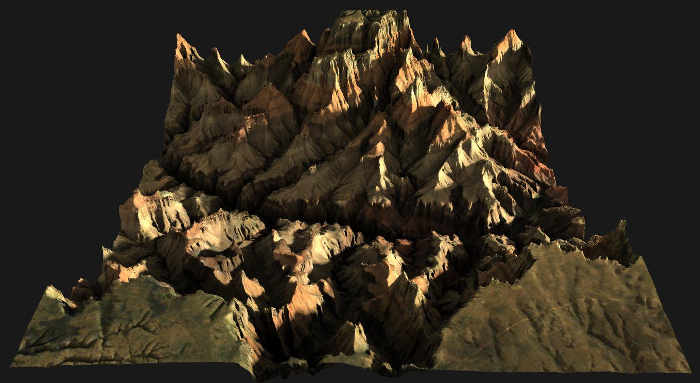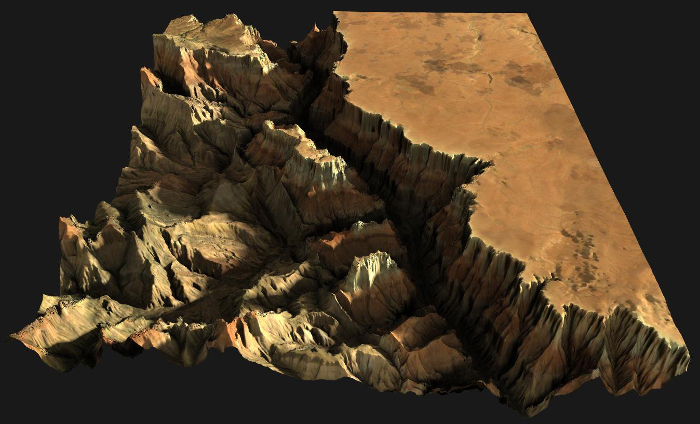Grand Canyon in 3D.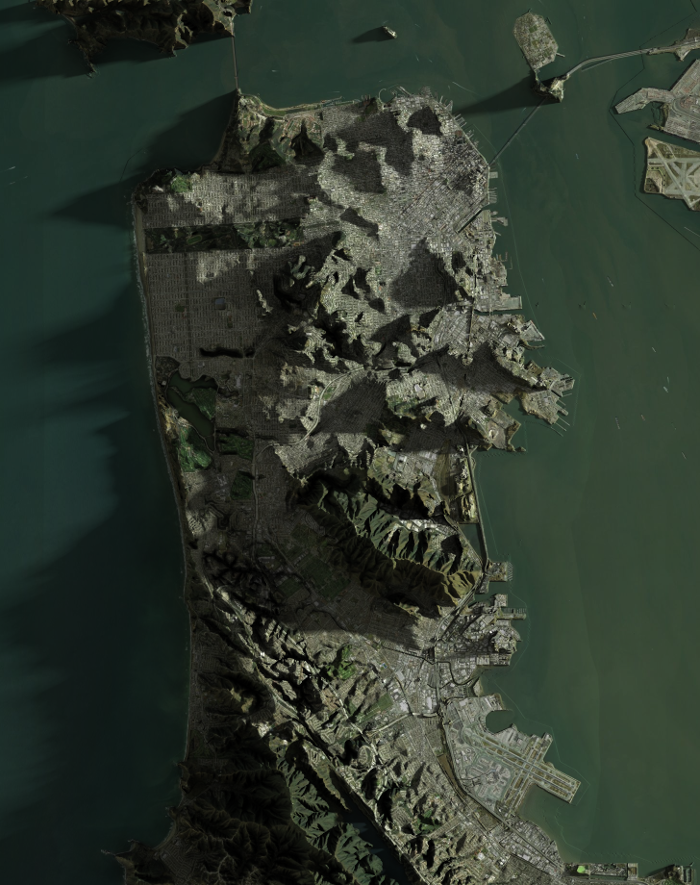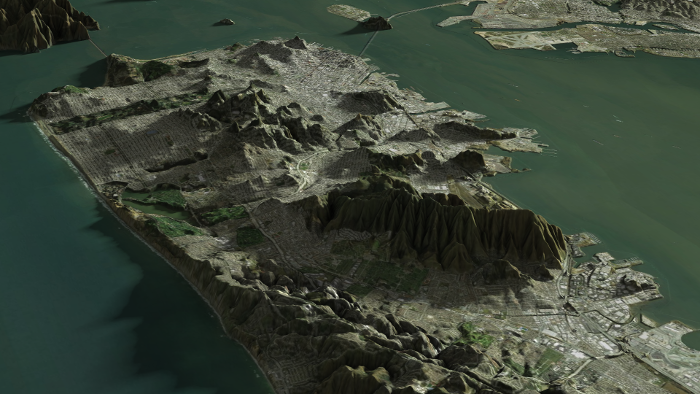San Francisco, 2D and 3D.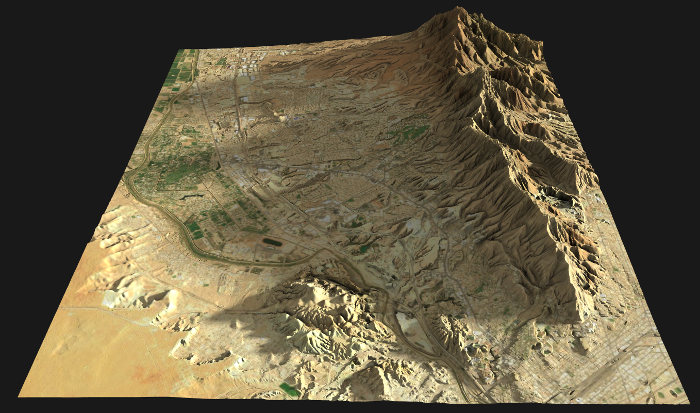My hometown, Westside El Paso.